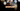# Leetcode | Solution of Fibonacci Number in JavaScript

April 1st, 2020
|

In this post, we will solve the problem Fibonacci number from leetcode and compute the time and space complexities. Let's begin.

# Problem Statement

The question can be found at leetcode Fibonacci number problem.

The problem states that given a number `N`, we need to return the `Nth` number in Fibonacci series.

# Fibonacci Series

The series starts with `0`, followed by `1` and the following numbers are the summation of last two numbers in the series

``example :-  0,1,1,2,3,5,8,13...so on``

# Solution

We can solve it using recursive or an iterative approach. For leetcode, we'll go ahead with an iterative approach. I'll link the repo down below if you want to check out the recursive approach.

The approach is simple

• We'll declare and initialize two variable `first` and `second` with values `0,1` respectively
• Next, we'll calculate the sum of those two
• Next, we'll iterate till `N` and keep swapping values i.e. assign the value in `second` to `first`, `sum` to `second` and `sum` would be the summation of new `first` and `second`
• This will ensure that the series keeps on moving for every iteration.
• once the loop exits, we return the last value i.e. the `sum`

We have discussed the approach, I urge you to go ahead on leetcode and give it another try.If you are here, it means something went wrong in implementation or you are just too lazy. In any case, let's see a simple implementation of the above logic.
``````var fib = function (N) {
if (N === 0 || N === 1) return N;

let first = 0, second = 1;
let sum = first + second;

for (let i = 2; i < N; i++) {
first = second;
second = sum;

sum = first + second;
}

return sum
};``````

Let's look at the solution.

On line 2, we take care of cases when `N` is equal to `0` or `1`. Next, we assign `0` and `1` to `first` and `second` variables. Then, we find their `sum` and iterate till `N` and keep the series moving. Once we exit out of the loop we return the `sum` i.e. the value at Nth position in the series.

After submission, here's the result

``````Status: Accepted
Runtime: 48ms
Memory: 34MB``````

## Time and space complexity

### Time complexity

We are iterating `N` times, so, time complexity would be O(n)

### Space complexity

We are not using any extra space except for a couple of variables, thus, space complexity would be O(1).

# Summary

So, we solved the Fibonacci number problem and computed it's space and time complexity.

I hope you enjoyed solving this question. This is it for this one, complete source code for this post can be found on my Github Repo. Will see you in the next one.